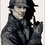# $\LaTeX$ Instructions

Some special and Important codes in $\LaTeX$ .

• \therefore appear as $\therefore$

• \gg appear as $\gg$

• \preceq appear as $\preceq$

• \doteq appear as $\doteq$

• \asymp appear as $\asymp$

• \ni appear as $\ni$

• \measuredangle appear as $\measuredangle$

• \dashv appear as $\dashv$

• \succ appear as $\succ$

• \ll appear as $\ll$

• \ast appear as $\ast$

• \star appear as $\star$

• \bigstar appear as $\bigstar$

• \square appear as $\square$

• \diamond appear as $\diamond$

• \triangle appear as $\triangle$

• \dagger appear as $\dagger$

• \uplus appear as $\uplus$

• \vee appear as $\vee$

• \wedge appear as $\wedge$

• \text{2}_2 appear as $\text{2}_2$

• \text{2}^2 appear as $\text{2}^2$

• \bullet appear as $\bullet$

• \wr appear as $\wr$

• \neq appear as $\neq$

• \langle appear as $\langle$

• \rangle appear as $\rangle$

• \top appear as $\top$

• \bot appear as $\bot$

• \emptyset appear as $\emptyset$

• \varnothing appear as $\varnothing$

• \sin appear as $\sin$

• \cos appear as $\cos$

• \tan appear as $\tan$

• \sec appear as $\sec$

• \csc appear as $\csc$

• \cosh appear as $\cosh$

• \pm appear as $\pm$

• \mp appear as $\mp$

• \alpha appear as $\alpha$

• \beta appear as $\beta$

• \backslash appear as $\backslash$

• \boxed{123} appear as $\boxed{123}$

• \sqrt{8} appear as $\sqrt{8}$

• \text{Matin is a Brilliant User} appear as $\text{Matin is a Brilliant User}$

• \dots appear as $\dots$

• \vdots appear as $\vdots$

• \LaTeX appear as $\LaTeX$

• \circ appear as $\circ$

• \huge{Brilliant is one} appear as $\huge{Brilliant~ is ~one}$

• \binom{8}{7} appear as $\binom{8}{7}$

• \cong appear as $\cong$

• \sim appear as $\sim$

• \models appear as $\models$

• \simeq appear as $\simeq$

• \diamondsuit appear $\diamondsuit$

• \bowtie appear as $\bowtie$

• \Im appear as $\Im$

• \infty appear as $\infty$

• \propto appear as $\propto$

• \mid appear as $\mid$

• \swarrow appear as $\swarrow$

• \nwarrow appear as $\nwarrow$

• \sewarrow appear as $\searrow$

• \nearrow appear as $\nearrow$

• \leadsto appear as $\leadsto$

• \rightleftharpoons appear as $\rightleftharpoons$

• \ddagger appear as $\ddagger$

• \mathcal{text} appear as $\mathcal{Matin}$

• \mathfrak{Matin} appear as $\mathfrak{Matin}$

• For mention some one use (@) and username like @Matin Naseri

• \frac{1}{2} appear as $\frac{1}{2}$

• \heartsuit appear as $\heartsuit$

* Text * appear as Text

• [text of link ] (Address of Link ) appear as Matin's profile

Ask your question or share other codes in $\LaTeX$Note by Matin Naseri
3 years, 3 months ago

This discussion board is a place to discuss our Daily Challenges and the math and science related to those challenges. Explanations are more than just a solution — they should explain the steps and thinking strategies that you used to obtain the solution. Comments should further the discussion of math and science.

When posting on Brilliant:

• Use the emojis to react to an explanation, whether you're congratulating a job well done , or just really confused .
• Ask specific questions about the challenge or the steps in somebody's explanation. Well-posed questions can add a lot to the discussion, but posting "I don't understand!" doesn't help anyone.
• Try to contribute something new to the discussion, whether it is an extension, generalization or other idea related to the challenge.
• Stay on topic — we're all here to learn more about math and science, not to hear about your favorite get-rich-quick scheme or current world events.

MarkdownAppears as
*italics* or _italics_ italics
**bold** or __bold__ bold
- bulleted- list
• bulleted
• list
1. numbered2. list
1. numbered
2. list
Note: you must add a full line of space before and after lists for them to show up correctly
paragraph 1paragraph 2

paragraph 1

paragraph 2

[example link](https://brilliant.org)example link
> This is a quote
This is a quote
    # I indented these lines
# 4 spaces, and now they show
# up as a code block.

print "hello world"
# I indented these lines
# 4 spaces, and now they show
# up as a code block.

print "hello world"
MathAppears as
Remember to wrap math in $$ ... $$ or $ ... $ to ensure proper formatting.
2 \times 3 $2 \times 3$
2^{34} $2^{34}$
a_{i-1} $a_{i-1}$
\frac{2}{3} $\frac{2}{3}$
\sqrt{2} $\sqrt{2}$
\sum_{i=1}^3 $\sum_{i=1}^3$
\sin \theta $\sin \theta$
\boxed{123} $\boxed{123}$

## Comments

Sort by:

Top Newest

I have a similar LaTex guide too. :)

- 3 years, 3 months ago

Log in to reply

Good.

I tried that collect all important codes in $\LaTeX$ into this discussion

- 3 years, 3 months ago

Log in to reply

you should add

\epsilon

which shows up as

$\epsilon$

- 2 years, 8 months ago

Log in to reply

like it.... $\heartsuit$

- 3 years, 3 months ago

Log in to reply

$\displaystyle \mathcal{Nice} \ \mathfrak{Note!}$

- 2 years, 8 months ago

Log in to reply

×

Problem Loading...

Note Loading...

Set Loading...